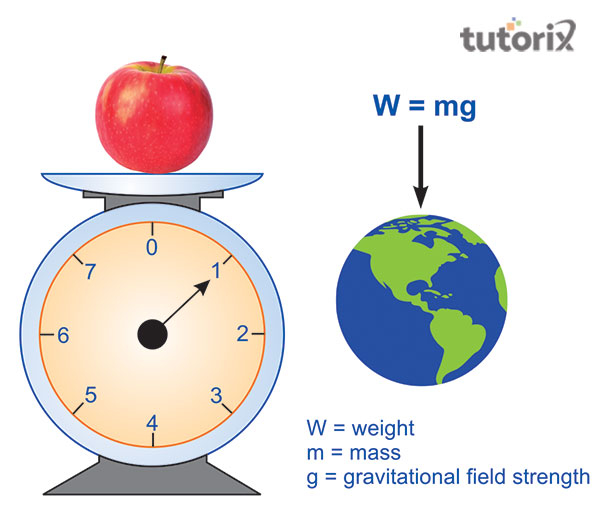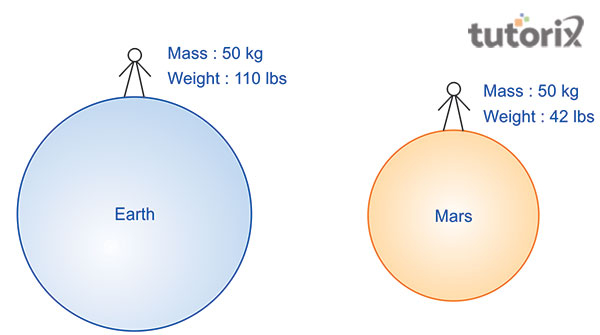# Difference Between Mass and Weight

## Introduction

Mass and weight both have differences and mass is a scalar quantity but weight is a vector quantity. It is seen that mass and weight are also correlated. The bodies having large mass also have a large weight. It is tough to through a large object because it has a large weight. The weight of a body is directly proportional to its mass. Here the matter is that weight of an object changes with the change of location.

## Definition of Mass

Mass is the quantity of matter possessed by a body. It is called a scalar quantity and it has only magnitude. The mass contains the SI unit and it is kilogram (K). Mass do not change with the change of location. The calculation of mass is done by using the formula, m = w/g. Different aspects like electronic balance, beam balance, and physical balance help in ten measurements of mass.

## Definition of Weight

Weight is the force of gravity and it is seen that this force attracts the Earth. Weight here is the vector quantity of an object. It has the SI unit and it is Newton (N). Although it is seen that weight and mass both are physical quantities, they have differences. Weight is referred to as the gravitational force exerted by the Earth. It seems equal to the product of the mass of the object and the value of the local gravitational acceleration. Weight is seen as proportional to the mass of the object. It has the sense, direction, and intensity and that is why it is called a vector quantity. It is also seen that weight is a variable value.Figure 1: Weight

The formula for weight is w=mg which helps in the measurement of the weight of an object. Weight is ensured using spring balance. The important idea here is that the measurement of weight can help to get the measurement of mass. Weight also varies from location to location as it can see that weight varies from earth to moon. The weight of an object on the earth will not be the same as that on the moon.

### Differences between Mass and WeightFigure 2: Difference between mass and weight

Sl No Matter of comparison Mass Weight
1. Definition

It is the measurement of the amount of matter within a body.

Weight refers to the measurement of the amount of force which acts on mass for the pull of gravity.

2. Meaning

Mass is the measurement of inertia

Here, weight is the measurement of force

3. Physical quantity

It is mainly a scalar quantity ad it has magnitude.

Weight, on the other hand, is a vector quantity. It has got the magnitude and it directs toward the centre of Earth as well as other gravity (Oxscience, 2022).

4. Location

Mass does not change as per the location and it remain the same everywhere.

The weight of an object varies from location to location.

5. SI unit

SI unit of mass is the kilogram (kg)

SI unit if the weight is Newton(N)

6. Gravitational force

The gravitational effect of mass can never be zero.

It can be zero when no gravity is acting on it.

7. Formula

The calculation of mass is done by using the formula, mass = volume × density.

The weight can be calculated using the formula, weight = mass × acceleration due to gravity.

8. Denotation

M is applied for the denotation of mass.

Using W does the denotation of weight.

9. Measurement Mass is measured by applying an ordinary balance such as beam balance, pan balance, lever balance and things like this (Greendale et al. 2019). Weight is measured using spring balance.

Table 1: Difference between Mass and Weight

### Measurement of Mass and Weight

There are different options for measuring mass and weight. A balanced is applied for determining the mass of an object. It is seen that the measurement is done by comparing the unknown mass of a body o a known estimate (Rossi et al. 2019). Weight is considered to be the influence of gravity and the formula for it is, W = m × g. Here, g refers to the acceleration due to gravity, m refers to mass and mass is expressed through Newtons (N).

## Conclusion

Mass and Weight are two different matters in this part. Weight is the amount of force whereas mass is the amount of matter within the object. The main difference between them says ‘that weight is the measurement of force and mass is the measurement of inertia. They also have different effects as per the location of the object. Mass remains the same in every location whereas the weight of an object changes as per the location. It is seen that both have different physical quantities and have different gravitational effects.

## FAQs

Q1. What is the definition of mass?

Ans. Mass is the measurement of the amount of matter that is present in the body of an object. It mainly means the measurement of inertia. Mass does not change with the application of force and a short portion is cut from the object.

Q2. What is the definition of weight?

Ans. Weight is nothing but the measurement of the amount of force. This force acts on mass due to the pull of gravity. It is seen that the unit of weight is equal to the unit of force. It is seen that weight is directly related to mass.

Q3. What instruments are used for measuring mass?

Ans. Some ordinary balance is used for the measurement of mass. Some of them are lever balance, beam balance, pan balance and so on.

Q4. What is used to denote mass and weight?

Ans. The denotation of weight is happened with the use of W. in the same way, M is used for the denotation of mass.

Updated on: 22-Aug-2023

257 Views